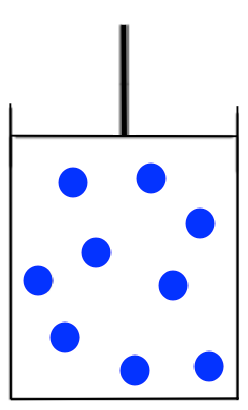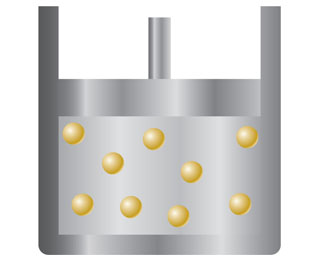# Problem: You have a sample of gas in a container with a movable piston, such as the one in the drawing.Redraw the container to show what it might look like if the temperature of the gas decreases from 300 to 200 K while the pressure is kept constant (assume the gas does not liquefy).

###### FREE Expert Solution

We are asked to redraw the given container to show what it might look like if the temperature of the gas decreases.Recall that Charles Law states that volume and temperature are directly proportional if pressure and moles are constant (i.e. As the volume goes up, the temperature also goes up, and vice-versa):

82% (318 ratings)###### Problem Details

You have a sample of gas in a container with a movable piston, such as the one in the drawing.Redraw the container to show what it might look like if the temperature of the gas decreases from 300 to 200 K while the pressure is kept constant (assume the gas does not liquefy).

Frequently Asked Questions

What scientific concept do you need to know in order to solve this problem?

Our tutors have indicated that to solve this problem you will need to apply the The Ideal Gas Law Applications concept. You can view video lessons to learn The Ideal Gas Law Applications. Or if you need more The Ideal Gas Law Applications practice, you can also practice The Ideal Gas Law Applications practice problems.